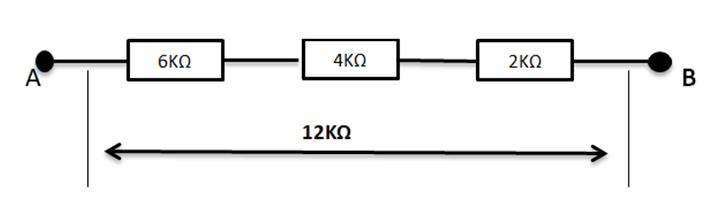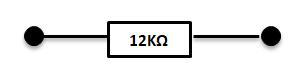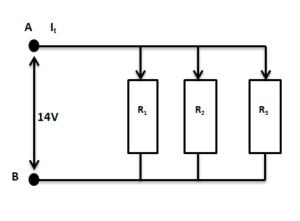Courses

# Combination of Resistors in Series and Parallel Class 12 Notes | EduRev

## Class 12 : Combination of Resistors in Series and Parallel Class 12 Notes | EduRev

The document Combination of Resistors in Series and Parallel Class 12 Notes | EduRev is a part of Class 12 category.
All you need of Class 12 at this link: Class 12

## Introduction

Resistors are two-terminal devices. Therefore, voltage division, regulation of current in the device and adjusting signal level are the functionality of a resistor. Representation of a resistor is done through Ohm’s Law.

R = VI

Many types of resistors are available and some are the following:

1. Wire-wound resistor.
2. Semi-conductor resistor.
3. Flim resistor.
4. Carbon Composition resistor.

## Resistor in Series

In this kind of connection, resistors are in a sequential array of resistors to form an electronic circuit/ device. Resistors are connected is in a single line and hence common current flows in the circuit.

The connection is in such a manner that the current flowing through the 1st register has to then flow further through the 2nd register and then through 3rd. Therefore, a common current is flowing in connection with a resistor in series. At all point in the circuit, the current amoung the resistors is same. For example,

I= I= I= It = 2ma

All the resistors in series that is R1, R2, R3 have current I1, I2, I3 respectively and the current of the circuit is It.

As resistors are connected in series the sum of the individual resistor is equal to the total resistance of the circuit. Let R1, R2, R3 be the resistors connected in series and Rtbe the total resistance of the circuit. so the total resistance of the circuit that is 12Ω, is the sum of all individual resistors R1, R2, R3 having 6KΩ, 4KΩ, 2KΩ respectively.This circuit of the resistors in series can also be represented byTherefore, the total resistance can be calculated as

R1 + R2 + R3 = Rt

furthermore, the total resistance of the above resistors in series is given by

Rt = 6KΩ + 4KΩ + 2KΩ = 12KΩ

### The Equation of Resistors in Series

Since the connection of resistor is in a series fashion that is in the sequential array or continuously one after other. The total resistance is equal to the resistance value of each resistor in the device/ circuit.

R1+R2+R3+R4+………………….Rn=Rt

where R is the resistance of the resistor and Rn represents the resistor number or the total resistance value.

## Resistor in Parallel

In this kind of connection, the terminals of resistors are connected to the same terminal of the other resistor to form an electronic circuit/ device. Resistors are connected is in parallel fashion and hence common voltage drop in the circuit.

Unlike, series connection, in parallel connection, current can have multiple paths to flow through the circuit, hence parallel connection is also current dividers. Common voltage drop is across the parallelly connected circuits/networks. At the terminals of the circuit, the voltage drop is always the same. For example

VR1=VR2=VR3=VRT=14V

The voltage across R1 is equal to the voltage across R2 and similarly, equal to R3 and hence the total voltage drop is equal to the voltage across the circuit. Reciprocal of individual resistance of each resistor and the sum of all the reciprocated resistance of resistor will us the total resistance of the circuit.

1(Rt) = 1(R1) + 1(R2) + 1(R3) +………… 1(Rn)Offer running on EduRev: Apply code STAYHOME200 to get INR 200 off on our premium plan EduRev Infinity!

,

,

,

,

,

,

,

,

,

,

,

,

,

,

,

,

,

,

,

,

,

;